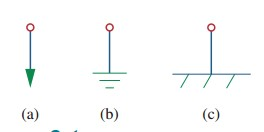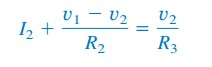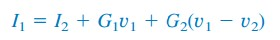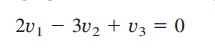omG8HI9LSKFW6lhCj8prTs0Z6lUhdOT9Jhi1Sf4m

# Nodal Analysis and Nodal Voltage Analysis in Electric Circuits

After learning about the fundamental laws of circuit theory (Ohm's law and Kirchhoff's laws), we will move on to electric circuits analysis.

Both Ohm's law and Kirchhoff's laws are not strong enough to analyze electric circuits with more complicated configuration.

What will we get from KVL or KCL for more complicated circuits with more complex branches, nodes, and elements? You will get a lot of equations you need to solve.

What will we do then? It will be time-consuming if we have to use elimination and substitution for more than 4 equations.

This is where we will use two powerful techniques for circuit analysis :
1. Nodal analysis
2. Mesh analysis
Make sure to read what is dc circuit first.

## Nodal Analysis Method

Nodal analysis needs us to calculate node voltages in each node with respect to the ground voltage (reference node), hence it is called the node-voltage method.

Nodal analysis is based on a systematic application of Kirchhoff's current law (KCL). With this technique, we will be able to analyze any linear circuit.Example of Nodal Analysis Circuits
What do you need to prepare before using this method? Keep in mind that we will get 2-n equations, where n is the number of elements and/or sources.

## Node Voltage Definition

Before moving on, let us define 'what is node voltage'. Node voltages are the potential difference between two nodes of a circuit.

Notice at the circuit above, where v1, v2, and v3 are the node voltages, connecting the corresponding node with element/s and another node. Not only that, but we also need to define a reference node (ground), hence this node is always called a ground node. Thus, this node voltage is 0 V.

## Nodal Analysis Theory

Nodal analysis is a technique using node voltages as main variables to analyze the electric circuits. If we only use the node voltages instead of element voltages as circuit variables, we will reduce the number of equations we need to solve greatly.

To lessen the difficulty in learning this technique, we will forbid the use of voltage source. Because we will treat voltage source in nodal analysis differently in the next post about Supernode Analysis.

In the nodal analysis, we are supposed to find node voltages. Given a circuit with n nodes without voltage sources, then we can use these three steps.
Steps to Determine Node Voltages :
1. Define a node as the reference node (ground) and give 0 V to it. Assign another node voltages v1, v2, ...., vn-1 to the remaining n - 1 nodes. The voltages are referenced with respect to the reference node.
2. Apply KCL for each n - 1 nonreference nodes. Use Ohm's law to find the current flowing in the branches.
3. Solve the resulting simultaneous equations to obtain the unknown node voltages.
Too short? Then let us read the full guide below throughout.

## Procedure of Nodal Analysis

The first step is to define a node as the reference or datum node or the ground node. The reference node is usually called the ground since it is assumed to have zero potential. The symbol of the reference node is shown in Figure.(1). Earth ground is shown in Figures.(1a) and (1b) and chassis ground is shown in Figure.(1c).Figure 1. Common symbols of ground
After we get the reference node, we assign voltage designations to reference nodes. Take a look at Figure.(2a) where node 0 is the reference node (v = 0), while nodes 1 and 2 are assigned with voltages v1 and v2 respectively.

Remember, node voltages are defined with respect to the reference node. As shown in Figure.(2a), each node voltage is the voltage rise from the reference node to the nonreference node or simply of the voltage node to the reference node.Figure 2. Typical circuit of nodal analysis
The second step is to apply KCL to each nonreference node in the circuit. To reduce the complexity of variables, Figure.(2a) is redrawn in Figure.(2b), where we use i1, i2, and i3 as the currents flowing through resistors R1, R2, and R3 respectively.

At node 1 we apply KCL and gives(1)
At node 2 gives(2)
We apply Ohm's law to express the unknown value of i1, i2, and i3 in terms of node voltages. Since the resistance is a passive element, using the passive sign convention, the current must always flow from higher potential to a lower potential.
Current flows from a higher potential to a lower potential in a resistor.
We can use this principle as,(3)
We obtain from Figure.(2b),(4)
Substituting Equations.(4) in (1) and (2) results(5)(6)
Substituting with conductances, Equations.(5) and (6) become(7)(8)
The third step is solving with node voltages. Applying KCL to n - 1 nonreference node, we obtain n - 1 simultaneous equation such as Equations.(5) and (6) or (7) and (8). For the circuit in Figure.(2) we solve Equations.(5) and (6) or (7) and (8) to get the node voltages v1 and v2 using any standard method such as substitution method, elimination method, Cramer's rule, or matrix inversion.

For example Equations.(7) and (8) will use matrix form as(9)
which can be solved to get v1 and v2.

## Nodal Analysis Examples

For better understanding, let us review some examples below :
1.Calculate the node voltages in the circuit in Figure.(3a)Figure 3
Solution :
Consider Figure.(3b) where the circuit in Figure.(3a) has been prepared for nodal analysis. The currents have been selected for KCL except for the branches with current sources.

The labelling of the current is arbitrary but consistent. (Consistent means if, for example, i2 enters the 4 Ω resistor from the left-hand side, i2 must leave the resistor from the right-hand side).

The reference node is selected and the node voltages v1 and v2 are now determined.
At node 1, applying KCL and Ohm's law gives
Multiplying each term in the last equation by 4, we obtain
or(1.1)
At node 2, we do the same and get
Multiplying each term by 12 results
or(1.2)
Now we have two simultaneous (1.1) and (1.2) and then we can solve using any method to get v1 and v2.
Method 1
Using elimination method we add (1.1) and (1.2) gives
Subsituting the result above with (1.1) gives
Method 2
Use Cramer's rule, we put (1.1) and (1.2) to matrix form as
The determinant is
We now obtain the voltages as
2.Determine the voltages at the nodes in Figure.(4a)
Solution :
In this example we will need three nonreference nodes instead of only two. We assign three nodes as can be seen in Figure.(4b)

At node 1,
Multiplying by 4 and rearranging terms, we get(2.1)
At node 2,
Multiplying by 8 and rearranging terms,we get(2.2)
At node 3,
Multiplying by 8, rearranging terms, and dividing by 3, we get(2.3)
We now will use elimination method, we add (2.1) and (2.3)(2.4)(2.5)
Substituting (2.5) into (2.4) we get
From (2.3), we get

Have you understood what is nodal analysis? Don't forget to share and subscribe! Happy learning!

Reference:  Fundamentals of electric circuits by Charles K. Alexander and Matthew N. O. Sadiku

Untuk Bahasa Indonesia baca Analisis Node
Related Posts
SHARE